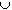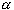#Interactive Real Analysis

## 5. Topology

### 5.2. Compact and Perfect Sets

We have already seen that all open sets in the real line can be written as the countable union of disjoint open intervals. We will now take a closer look at closed sets. The most important type of closed sets in the real line are called compact sets:
 Definition 5.2.1: Compact Sets A set S of real numbers is called compact if every sequence in S has a subsequence that converges to an element again contained in S.
 Examples 5.2.2:Is the interval [0,1] compact ? How about [0, 1) ?Is the set {1, 2, 3} compact ? How about the set N of natural numbers ?Is the set {1, 1/2, 1/3, 1/4, ...} compact ?Is the set {1, 1/2, 1/3, 1/4, ...}{0} compact ?
It is not easy to see what compact sets really look like, based on this definition. However, the following result gives a nice characterization of them, and lets you answer the above questions easily.
 Proposition 5.2.3: Compact means Closed and Bounded A set S of real numbers is compact if and only if it is closed and bounded.
The above definition of compact sets using sequence can not be used in more abstract situations. We would also like a characterization of compact sets based entirely on open sets. We need some definitions first.
 Definition 5.2.4: Open Cover Let S be a set of real numbers. An open cover of S is a collection C of open sets such that SC. The collection C of open sets is said to cover the set S. A subset of sets from the collection C that still covers the set S is called a subcovering of S.
 Examples 5.2.5:Let S = [0, 1], and C = { (-1/2, 1/2), (1/3, 2/3), (1/2, 3/2)}. Is C an open cover for S ?Let S = [0, 1]. Define= { tR : | t -| <andS} for a fixed> 0. Is the collection of all {},S, an open cover for S ? How many sets of typeare actually needed to cover S ?Let S = (0, 1). Define a collection C = { (1/j, 1), for all j > 0 }. Is C an open cover for S ? How many sets from the collection C are actually needed to cover S ?
Here is the characterization of compact sets based only on open sets:
 Theorem 5.2.6: Heine-Borel Theorem A set S of real numbers is compact if and only if every open cover C of S can be reduced to a finite subcovering.
Compact sets share many properties with finite sets. For example, if A and B are two non-empty sets with AB then AB # 0. That is, in fact, true for finitely many sets as well, but fails to be true for infinitely many sets.
 Examples 5.2.7:Consider the collection of sets (0, 1/j) for all j > 0. What is the intersection of all of these sets ?Can you find infinitely many closed sets such that their intersection is empty and such that each set is contained in its predecessor ? That is, can you find sets Aj such that Aj+1Aj andAj = 0 ?
Compact sets, on the other hand, have the following nice property, which will be used in some of the following chapters:
 Proposition 5.2.8: Intersection of Nested Compact Sets Suppose { Aj } is a collection of sets such that each Aj non-empty, compact, and Aj+1Aj. Then A =Aj is not empty.

Another interesting collection of closed sets are the perfect sets:

 Definition 5.2.9: Perfect Set A set S is perfect if it is closed and every point of S is an accumulation point of S.
 Example 5.2.10:Find a perfect set. Find a closed set that is not perfect. Find a compact set that is not perfect. Find an unbounded closed set that is not perfect. Find a closed set that is neither compact nor perfect.Is the set {1, 1/2, 1/3, ...} perfect ? How about the set {1, 1/2, 1/3, ...}{0} ?
As an application of the above result, we will see that perfect sets are closed sets that contain lots of points:
 Proposition 5.2.11: Perfect sets are Uncountable Every non-empty perfect set must be uncountable.

This can yield a quick, but rather sophisticated proof of the fact that the interval [a, b] is uncountable: the interval [a, b] is a perfect set, hence, it must be uncountable.

Another, rather peculiar example of a closed, compact, and perfect set is the Cantor set.

 Definition 5.2.12: Cantor Middle Third Set Start with the unit interval S0 = [0, 1] Remove from that set the middle third and set S1 = S0 \ (1/3, 2/3) Remove from that set the two middle thirds and set S2 = S1 \ { (1/9, 2/9)(7/9, 8/9) } Continue in this fashion, where Sn+1 = Sn \ { middle thirds of subintervals of Sn } Then the Cantor set C is defined as C =Sn
The Cantor set gives an indication of the complicated structure of closed sets in the real line. It has the following properties:
 Example 5.2.13: Properties of the Cantor SetShow that the Cantor set is compact (i.e. closed and bounded)Show that the Cantor set is perfect (and hence uncountable)Show that the Cantor set has length zero, but contains uncountably many points.Show that the Cantor set does not contain any open set